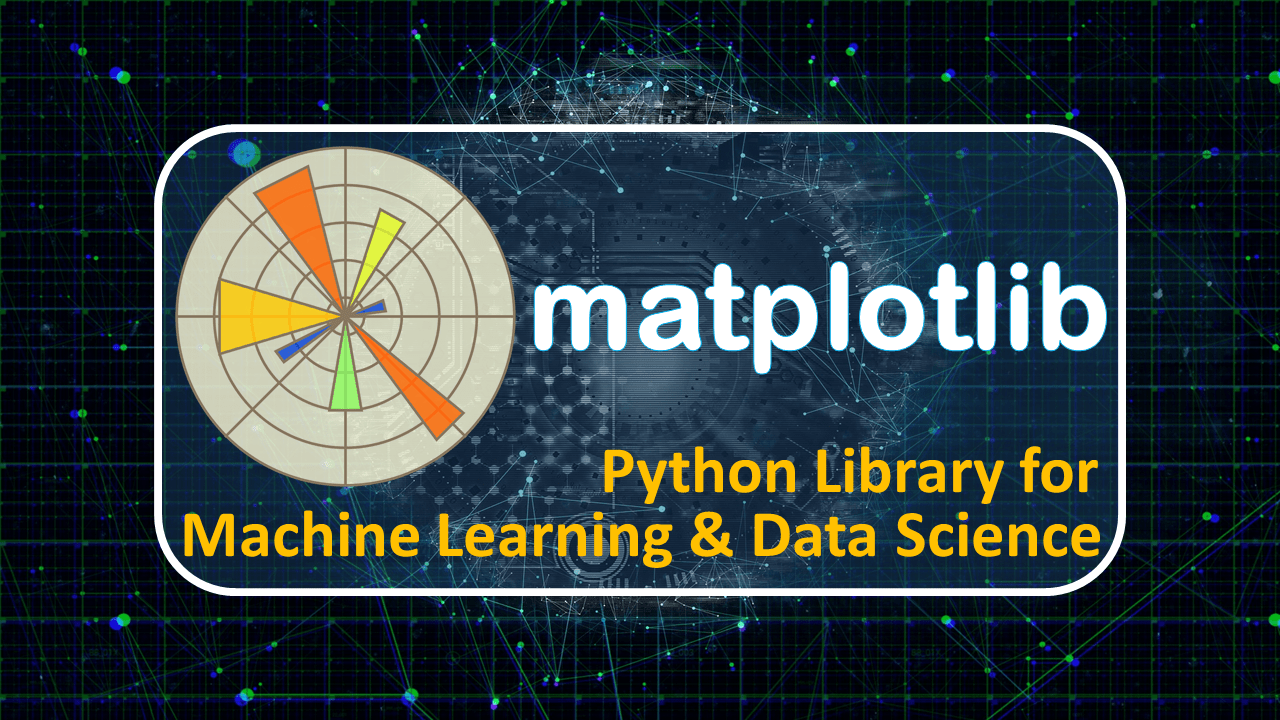## Matplotlib imshow – Read & Show image using imread() & plt.imshow()

If you worry about, how to read and show an image using the matplotlib library then here you will get a solution for your problem. Along with that, you will be got a bonus. The matplotlib imshow() function helps to show the image. But plt.imshow() didn't work without mpimg.imread() function which is belongs to matplotlib.image module. So lets start practical. Import Libraries import...## Matplotlib savefig – Matplotlib Save Figure | Python matplotlib Tutorial

Matplotlib Save Figure After creating a plot or chart using the python matplotlib library and need to save and use it further. Then the matplotlib savefig function will help you. In this blog, we are explaining, how to save a figure using matplotlib? Import Library import matplotlib.pyplot as plt # for data visualization Matplotlib SaveFig (save figure) Different ways Syntax: plt.savefig(    ...## Matplotlib Subplot – plt.subplot() | Python Matplolib Tutorial

Matplotlib Subplot This blog under construction but you can download sourse code In matplolib subplot blog, discusing on how to plot subplots using plt.subplot() function. Imort Libraries import matplotlib.pyplot as plt import numpy as np import pandas as pd import random from matplotlib import style Create matplotlib subplotls Creating 4 subplot with 2 rows and 2 columns. # Create 4 subplot (2-...## Matplotlib Scatter Plot – plt.scatter() | Python Matplotlib Tutorial

Matplotlib Scatter Plot In the matplotlib scatter plot blog will discuss, how to draw a scatter plot using python matplotlib plt.scatter() function. The plt.scatter() function help to plot two-variable datasets in point or a user-defined format. Here, we will be plotting google play store apps scatter plot. Import Libraries import matplotlib.pyplot as plt # For visualization import pandas as pd...## Matplotlib Pie Chart – plt.pie() | Python Matplotlib Tutorial

Matplotlib Pie Chart In this blog, we will work on how to draw a matplotlib pie chart? To draw pie char use plt.pie() function. The matplotkib plt.pie() function help to plot pie chart of given numeric data with labels. It also support different parameters which help to show better. Importing Library import matplotlib.pyplot as plt Plotting Matplotlib Pie Chart plt.pie( ) # Plot pie chart of...## Matplotlib Bar Chart – Python Matplotlib Tutorial

Matplotlib Bar Chart To visualize value associated with categorical data in the bar format use matplotlib bar chart plt.bar() or plt.barh() methods. Importing Libary to Plot Bar Chart import matplotlib.pyplot as plt import numpy as np from matplotlib import style Importing Data set to plot Bar Chart This dataset of "Indian Artificial Intelligence Production Class" (IAIP).  Instead of...## Matplotlib Histogram – Python Matplotlib Tutorial

Python Matplotlib Histogram Matplotlib histogram is a representation of numeric data in the form of a rectangle bar. Each bar shows some data,  which belong to different categories. To plot histogram using python matplotlib library need plt.hist() method. Syntax:...## Matplotlib Line Plot – Python Matplotlib Tutorial

Matplotlib Line Plot In this blog, you will learn how to draw a matplotlib line plot with different style and format. The pyplot.plot() or plt.plot() is a method of matplotlib pyplot module use to plot the line. Syntax: plt.plot(*args, scalex=True, scaley=True, data=None, **kwargs) Import pyplot module from matplotlib python library using import keyword and give short name plt using as ...## Python Matplotlib Tutorial – Mastery in Matplotlib Library

Python Matplotlib Tutorial After complication of python NumPy Tutorial and python pandas tutorial. Now, we jump on the python matplotlib tutorial to become a master in it. What is python matplotlib? Matplotlib is a 2D and 3D graph plotting python library. It also supports to create animations and images. This is an advanced part of python matplotlib.  Using python matplotlib, you can draw a...
Top